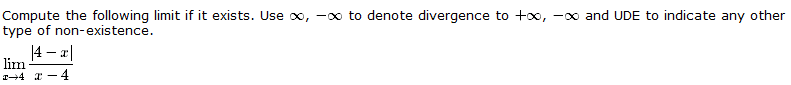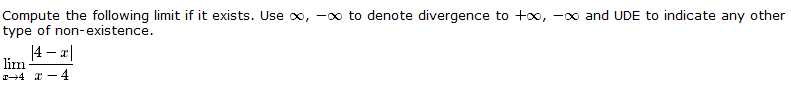# Find the limit question: Kinda confusing.

• nukeman
Please try again.Correct, the limit from the left doesn't equal the limit from the right, so the overall limit does not exist.Please try again.f

## Homework Statement

Image here shows the question:## The Attempt at a Solution

I thought the answer would be limit = infinity

I don't understand how to find the limit to this question.

## Homework Statement

Image here shows the question:## The Attempt at a Solution

I thought the answer would be limit = infinity

I don't understand how to find the limit to this question.

Try simplifying the expression |4 - x| / (x - 4). Consider two cases separately: x < 4 and x > 4.

Thats what I did. I got to x < 4 and x > 4

After that, what do I do?

isnt this undefined?

Thats what I did. I got to x < 4 and x > 4

After that, what do I do?

What is |4 - x| / (x - 4) when x < 4?

What is |4 - x| / (x - 4) when x > 4?

What is |4 - x| / (x - 4) when x < 4?

What is |4 - x| / (x - 4) when x > 4?

My results were -1

My results were -1

For both cases? If so, the limit would be -1.

However, you made a mistake in one of the cases.

For both cases? If so, the limit would be -1.

However, you made a mistake in one of the cases.

I did?

I did?

You did, if you got -1 for both cases.

What does |4 - x| simplify to, if x > 4?
What does |4 - x| simplify to, if x < 4?

What does |4 - x| simplify to, if x > 4?
What does |4 - x| simplify to, if x < 4?

I am sorry, I don't understand :(

I think what is messing me up is the absolute value bars

The absolute value function is defined as:

|a| = -a if a<0
|a| = a if a>=0

This is what you have to work with.

Ahhh...So, then my calc comes out as -1 and 1, therefore it does not equal so its undefined?

Ahhh...So, then my calc comes out as -1 and 1, therefore it does not equal so its undefined?

Correct, the limit from the left doesn't equal the limit from the right, so the overall limit does not exist.